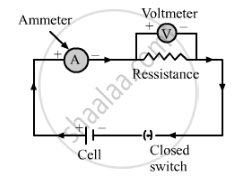Share
Notifications

View all notifications
Books Shortlist
Your shortlist is empty

# Draw the Labelled Diagram of an Electric Circuit Comprising of a Cell, a Resistance, an Ammeter, a Voltmeter and a Closed Switch (Or Closed Plug Key). Which of the Two Has a Large Resistance : an Ammeter Or a Voltmeter? - CBSE Class 10 - Science

Login
Create free account

Forgot password?

#### Question

Draw the labelled diagram of an electric circuit comprising of a cell, a resistance, an ammeter, a voltmeter and a closed switch (or closed plug key). Which of the two has a large resistance : an ammeter or a voltmeter?

#### Solution

Here is the diagram of an electric circuit comprising a cell, a resistance, an ammeter, a voltmeter and a closed switchA voltmeter has a high resistance so that it takes only a negligible amount of current from the circuit.

Is there an error in this question or solution?

#### APPEARS IN

Solution Draw the Labelled Diagram of an Electric Circuit Comprising of a Cell, a Resistance, an Ammeter, a Voltmeter and a Closed Switch (Or Closed Plug Key). Which of the Two Has a Large Resistance : an Ammeter Or a Voltmeter? Concept: Circuit Diagram.
S